×#### Thank you for registering.

One of our academic counsellors will contact you within 1 working day.

Click to Chat

1800-1023-196

+91-120-4616500

CART 0

• 0

MY CART (5)

Use Coupon: CART20 and get 20% off on all online Study Material

ITEM
DETAILS
MRP
DISCOUNT
FINAL PRICE
Total Price: Rs.

There are no items in this cart.
Continue Shopping• Complete JEE Main/Advanced Course and Test Series
• OFFERED PRICE: Rs. 15,900
• View Details

```Chapter 3: Functions – Exercise 3.2

Functions – Exercise 3.2 – Q.1

We have,

f(x) = x2 - 3x + 4

Now,

f(2x + 1) = (2x + 1)2 - 3(2x + 1) + 4

= 4x2 + 1 + 4x - 6x - 3 + 4

= 4x2 - 2x + 2

It is given that

f(x) = f(2x + 1)

⟹ x2 — 3x + 4 = 4x2 - 2x + 2

⟹ 0 = 4x2 - x2 - 2x + 3x + 2 - 4

⟹ 3x2 + x - 2 = 0

⟹ 3x2 + 3x - 2x - 2 = 0

⟹ 3x (x + 1) - 2 (x + 1) = 0

⟹ (x +1) (3x -2) = 0

⟹ x + 1 = 0   or    3x - 2 = 0

⟹ x = -1 or x = 2/3

Functions – Exercise 3.2 – Q.2

We have,

f(x) = (x – a)2 (x – b)2

Now,

f(a + b) = (a + b – a)2 (a + b – b)2

= b2a2

⟹ f(a + 6) = a2b2

Functions – Exercise 3.2 – Q.3

We have,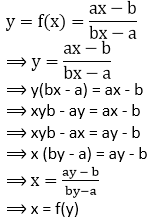Hence, proved

Functions – Exercise 3.2 – Q.4

We have,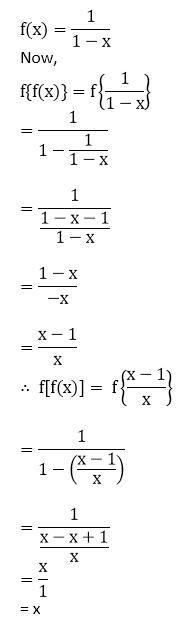∴ f[f(x)] = x Hence, proved

Functions – Exercise 3.2 – Q.5

We have,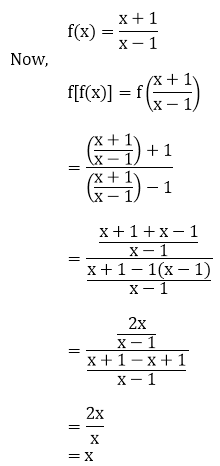∴  f[f(x)] = x Hence, proved

Functions – Exercise 3.2 – Q.6

We have,Functions – Exercise 3.2 – Q.7

We have,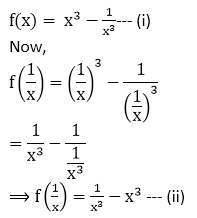Adding equation (i) and equation (ii), we get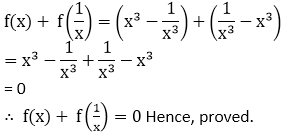Functions – Exercise 3.2 – Q.8

We have,∴ f(tan θ) = sin 2θ Hence, proved.

Functions – Exercise 3.2 – Q.9

We have,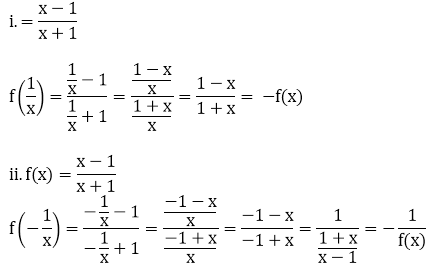Functions – Exercise 3.2 – Q.10

We have,

f(x) = (a - xn)1/n, a > 0

Now,

f{f(x)} = f(a - xn)1/n

= [a-{(a - xn)1/n}n]1/n

= [a - (a - xn)]1/n

= [a - a + xn]1/n

= (xn)1/n

= (x)n×1/n

= x

∴ f{f(x)} = x Hence, proved.
```### Course Features

• 728 Video Lectures
• Revision Notes
• Previous Year Papers
• Mind Map
• Study Planner
• NCERT Solutions
• Discussion Forum
• Test paper with Video Solution